NEET  >  DC Pandey Solutions: Electromagnetic Induction - 1

# DC Pandey Solutions: Electromagnetic Induction - 1 - Notes | Study Physics Class 12 - NEET

 1 Crore+ students have signed up on EduRev. Have you?

Introductory Exercise 24.1

Ques 1: Figure shows a conducting loop placed near a long straight wire carrying a current i as shown. If the current increases continuously, find the direction of the induced current in the loop.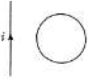Sol:
magnetic field passing through loop is increasing. Hence induced current will produce magnetic field. So, induced current should be anti-clockwise.

Ques 2: A metallic loop is placed in a nonuniform steady magnetic field. Will an emf be induced in the loop?
Sol:
It is true that magnetic flux passing through the loop is calculated by integration. But it remains constant.

Ques 3: Write the dimensions of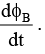Sol: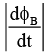=  [Potential or EMF]
= [ML2A-1T-3]

Introductory Exercise 24.2

Ques 1: A triangular loop is placed in a dot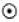magnetic field as shown in figure. Find the direction of induced current in the loop if magnetic field is increasing.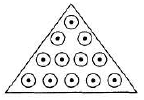Sol:is increasing. Henceis produced by the induced current. So, it is clockwise.

Ques 2: A rectangular loop is placed near a current carrying straight wire as shown in figure. If the loop is rotated about an axis passing through one of its sides, find the direction of induced current in the loop.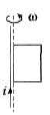Sol:
Magnetic lines around the straight wire are circular. So, same magnetic lines will pass through loop under all conditions.
ΔΦ = 0 ⇒    emf = 0 ⇒ i = 0

Ques 3: Two circular loops lie side by side in the same plane. One is connected to a source that supplies an increasing current, the other is a simple closed ring. Is the induced current in the ring is in the same direction as that in the loop connected to the source or opposite? What if the current in the first loop is decreasing?
Sol:
By increasing the current in loop-1 magnetic field in ring-2 in downward direct ion will increase. Hence induced current in ring-2 should produce upward magnetic field. Or current in ring should be in the same direction.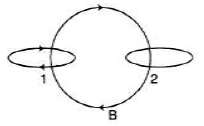Introductory Exercise 24.3

Ques 1: A loop of wire enclosing an area S is placed in a region where the magnetic field is perpendicular to the plane. The magnetic field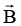varies wit h time according to the expression B = B0e-at where a is so me constant. That is, at t = 0. The field is B0 and for t > 0, the field decreases exponentially. Find the induced emf in the loop as a function of time.
Sol: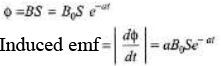Ques 2: As the bar shown in figure moves in a direct ion perpendicular to the field, is an external force required to keep it moving with constant speed.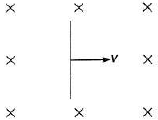Sol: Circuit is not closed. So, current is zero or magnetic force is zero.

Ques 3: A coil formed by wrapping 50 turns of wire in the shape of a square is positioned in a magnetic field so that the normal to the plane of the coil makes an angle of 30°, with the direction of the field. When the magnetic field is increased uniformly from 200 μT to 600 μT in 0.4 s, an emf of magnitude 80.0 mV is induced in the coil. What is the total length of the wire?
Sol: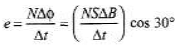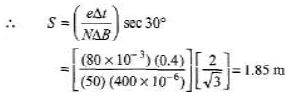Side of square = 1.36 m Total length of wire = 50 (4 × 1.36) = 272 m

Ques 4: The long straight wire in figure (a) carries a constant current i. A metal bar of length l is moving at constant velocity v as shown in figure. Point a is a distance d from the wire.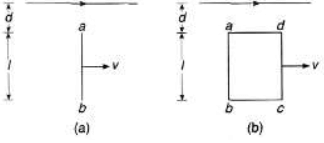(a) Calculate the emf induced in the bar.
(b) Which point a or b is at higher potential?
(c) If the bar is replaced by a rectangular wire loop of resistance R, what is the magnitude of current induced in the loop?
Sol:
(a) At a distance x from the wire magnet ic field over the wire ab is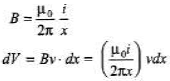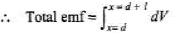(b) Magnetic field due to current i over the wire ab is inwards. Velocity of wire ab is towards right. Applying right hand rule we can see that a point is at higher potential.
(c) Net change in flux through the loop abcd is zero.
Hence induced emf is zero. So, induced current is zero.

The document DC Pandey Solutions: Electromagnetic Induction - 1 - Notes | Study Physics Class 12 - NEET is a part of the NEET Course Physics Class 12.
All you need of NEET at this link: NEET

## Physics Class 12

157 videos|452 docs|213 tests
 Use Code STAYHOME200 and get INR 200 additional OFF

## Physics Class 12

157 videos|452 docs|213 tests

### How to Prepare for NEET

Read our guide to prepare for NEET which is created by Toppers & the best Teachers

Track your progress, build streaks, highlight & save important lessons and more!

,

,

,

,

,

,

,

,

,

,

,

,

,

,

,

,

,

,

,

,

,

;